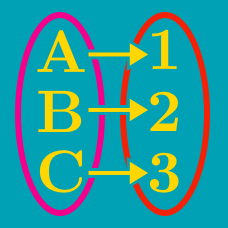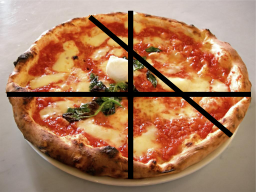Probability

# Pizza Numbers WarmupWhat is the maximum number of pieces you can divide this pizza into with a single cut?

Note: All cuts must be distinct straight lines from one point on the edge of the pizza to another point on the edge of the pizza.What is the minimum number of cuts necessary to divide this pizza into 5 pieces?

Note: All cuts must be distinct straight lines from one point on the edge of the pizza to another point on the edge of the pizza.Without folding or stacking pieces, is it possible to divide this pizza into 8 pieces with 3 cuts?

Note: All cuts must be distinct straight lines from one point on the edge of the pizza to another point on the edge of the pizza.Given that the pizza above has already been divided into 7 pieces with 3 cuts, what is the maximum number of pieces it could be divided into after one additional cut?

Note: All cuts must be distinct straight lines from one point on the edge of the pizza to another point on the edge of the pizza.Is it possible to divide this pizza into 6 pieces with 4 cuts?

Note: All cuts must be distinct straight lines from one point on the edge of the pizza to another point on the edge of the pizza.

×